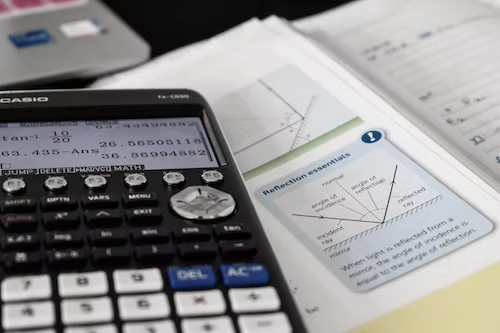## How Much Do Different Doctors Make?Are you considering a career in the medical field but unsure which specialization may be the right fit for you? We have compiled a list of average annual salaries for a variety of different physicians so you can compare each field and hopefully narrow down your options. Pediatric Physician – \$244,000 To become a pediatric physician, you will first need to complete four years of undergraduate schooling. Throughout undergraduate, you…

## How to Find Vertical Asymptotes?Vertical asymptotes are an essential concept in calculus and are used to describe the behavior of functions as they approach infinity. A vertical asymptote is a line that a curve approaches but never touches, as it tends to infinity in the y-axis. These lines occur when a function approaches a certain value, and its denominator approaches zero. As a result, it is crucial to learn how to find vertical asymptotes,…

## Intermediate Value TheoremThe intermediate value theorem states that for any value between the minimum and maximum values of a continuous function, there exists a corresponding input that produces that value as output. It supports two key statements: If a function is continuous on a closed interval [a,b], then for every y between f(a) and f(b), there exists a x in [a,b] such that f(x) = y. If a function is continuous on…

## What are Supplementary Angles?Angles are everywhere, whether we see them or not. Supplementary angles are just one of many types of angles out there that hold up our world. Unlike acute and obtuse angles, the term “supplementary” refers to not just one but several angles, all next to each other. For a set of angles to be supplementary, all angles must add up to 180 degrees. Supplementary angles can be made of both…

## An Introduction to Significant FiguresWhen you complete the math section of the SAT, you will see some questions requiring you to round your answer to a certain number of significant figures. If you don’t know what significant figures are, you may very well miss these questions, even if you have completed the right calculations to solve the math problem. To do well on standardized tests like the SAT or in your advanced math classes,…

## Mean, Median, Mode: What are they and how to find them?When you take the SAT or the ACT, you will encounter some statistics questions on the math sections. These questions typically ask you to find the mean, median, or mode of a data set. To earn a perfect score on the SAT or ACT Math sections, it is critical that you know the definitions of these terms as well as how to calculate them in a short amount of time.…

## How To Divide Fractions – A Complete GuideWhen you complete the math sections on the SAT or the ACT, you are going to need to know how to add, subtract, multiply, and divide fractions. There will likely be a couple of questions where you will have to divide fractions within a limited amount of time, meaning that you’ll need to know what fractions are and how to divide them with ease. Considering you only have 75 seconds…

## How To Calculate The Volume Of a SphereThe math sections on the SAT and ACT will require you to answer geometry related questions.  If you’re taking the test during your junior or senior year, chances are you may be a little rusty when it comes to geometry concepts you learned years before. Before test day, you’ll want to brush up on key formulas for calculating the area, circumference, and volume of different shapes, including spheres. While you…

## How To Multiply Fractions – A Complete GuideWhether you take the SAT or the ACT, you are going to need to be comfortable with multiplying fractions.  You will see many questions that require you to not only understand how fractions work, but also be able to multiply these fractions within a limited amount of time.  On the SAT Math sections, you will have 75 seconds per question for the Math (No Calculator) section, and 87 seconds per…